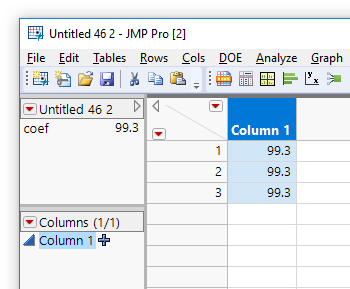Our World Statistics Day conversations have been a great reminder of how much statistics can inform our lives. Do you have an example of how statistics has made a difference in your life? Share your story with the Community!
Choose Language Hide Translation Bar
Highlighted

## How to pass a variable into a formula?

I am using the below syntax. I would like to modify one part of the formula which is the estimated MA coefficient.

In this case, the value of the MA Coefficient is 0.144654220611782.

My numerous attempts so far, includign using lists, have fallen flat.

How could I assign the coefficient value (of 0.144654220611782) to a "variable" and then use that "variable" in the formula? Meaning I assign the coefficient value before running the formula syntax, with the value passed into the formula in a suitable manner.

``````dt << New Column( "Yt Forecast1", Numeric, "Continuous", Format( "Best", 12 ),
Formula(
ARIMA Forecast(
:Yt,
50,
ARIMA( 0, 1, 1, No Intercept( 1 ) ),
{MA Coefficients( {0.144654220611782} )},
Row() - 50,
Row() - 50
)))``````
1 ACCEPTED SOLUTION

Accepted Solutions
Highlighted

## Re: How to pass a variable into a formula?

What has worked for me is this. I get the value "tempMACoeff " from the list from lstModel = ts << Get Models; (ts is the time series analysis)

The column formula accepts a new value for tempMACoeff and will update...

``````col = dt << New Column( "Yt-Forecast", Numeric);
dt << set Table Variable( "coef", tempMACoeff );
col << Set Formula( Eval(
Eval Expr(
ARIMA Forecast(
:Yt,
50,
ARIMA( 0, 1, 1, No Intercept( 1 ) ),
{MA Coefficients( {Expr( :coef )} )},//
Row() - 50,
Row() - 50
)
)
)); // set the formula``````

7 REPLIES 7
Highlighted

## Re: How to pass a variable into a formula?

Use a Table Variable, by scripting, or from the red triangle.

``````New Table( "Untitled 46",
New Table Variable( "coef", 99.3 ),
New Column( "Column 1",
Numeric,
"Continuous",
Format( "Best", 12 ),
Formula( :coef ),
Set Selected
)
)``````Table Variable

Craige
Highlighted

## Re: How to pass a variable into a formula?

You need something like this. When you change the Table Variable, the column updates.

``````Eval(
Eval Expr(
ARIMA Forecast(
:age,
40,
ARIMA( 0, 1, 1, No Intercept( 1 ) ),
{MA Coefficients( {Expr( :coef )} )},
Row() - 9,
Row() - 9
)
)
)``````

Using the same table variable idea, notice how the eval( eval expr( ... expr(:coef) ... ) ) works. expr(:coef) identifies a snippet of the formula that needs a substitution made by evaluating it. evalexpr(...) does the evaluation of all the bits identified with expr, and returns an expression that still needs to be evaluated. eval(...) does that. (I changed all the parameters to make it do something with bigclass...don't use mine! I'm not familiar with the ARIMA forecast functions, or if it should be used in a column formula like this. The scripting index example does not use a column formula.)

Craige
Highlighted

## Re: How to pass a variable into a formula?

Here is a slightly different way of handling the same solution as Craige provided.  The only difference is that this version hard codes the Coef value into the formula, not requiring a Table Variable

``````Names Default To Here( 1 );
dt = Current Data Table();
coef = 99.3;
Eval(
Substitute(
Expr(
New Table( "the table",
New Table Variable( "coef", 99.3 ),
New Column( "Column 1",
Numeric,
"Continuous",
Format( "Best", 12 ),
Formula( __coef__ ),
Set Selected
)
)
),
Expr( __coef__ ), coef
)
);``````
Jim
HighlightedDavid_Burnham
Super User

## Re: How to pass a variable into a formula?

Another approach is to convert the formula to a string representation and then do standard string substitution, converting it back to an expression when you assign the column formula:

``````strFml = Char(Expr(

ARIMA Forecast(
:Yt,
50,
ARIMA( 0, 1, 1, No Intercept( 1 ) ),
{MA Coefficients( {_coeff_} )},
Row() - 50,
Row() - 50
)

));;

coeffValue = 0.144;
SubstituteInto("_coeff_",coeffValue);

dt << New Column("Y Forecast", numeric, Set Formula( Eval(Parse(strFml)) ));``````
-Dave
Highlighted

## Re: How to pass a variable into a formula?

Dave, I wasn't able to get this to work out. I resorted to the below which seems to the job I'm hoping to get done.
Thanks, Scott.
Highlighted

## Re: How to pass a variable into a formula?

What has worked for me is this. I get the value "tempMACoeff " from the list from lstModel = ts << Get Models; (ts is the time series analysis)

The column formula accepts a new value for tempMACoeff and will update...

``````col = dt << New Column( "Yt-Forecast", Numeric);
dt << set Table Variable( "coef", tempMACoeff );
col << Set Formula( Eval(
Eval Expr(
ARIMA Forecast(
:Yt,
50,
ARIMA( 0, 1, 1, No Intercept( 1 ) ),
{MA Coefficients( {Expr( :coef )} )},//
Row() - 50,
Row() - 50
)
)
)); // set the formula``````

Highlighted

## Re: How to pass a variable into a formula?

Column formulas subscribe to other columns and table variables to listen for changes and reevaluate when that happens.  Not to other JSL variables though. So, the way it works for you, the formula can see the coef variable and knows it should subscribe for changes. I think the other suggestions are replacing the variable with a constant, so the formula no longer knows it should subscribe to something.

Craige
Article Labels

There are no labels assigned to this post.